Exercises

# Multiplication 1

Third grade is the year of multiplication. Though it was most likely introduced to kids in second grade, third grade is when kids are tasked with mastering their times tables and developing a stronger understanding of this key operation. This guided lesson in understanding multiplication can help give third graders a leg up. For even more practice, consider downloading the recommended multiplication worksheets that accompany the lesson.
This lesson includes 5 printable learning activities.

## Skip Counting at the Zoo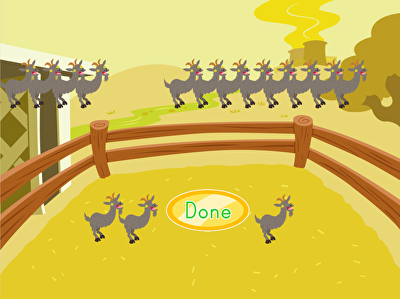Something is not right at this petting zoo. In Skip Counting at the Zoo, third graders get practice with skip counting as they drag and drop mutated animals into a pen to match the numbers. Some animals have one leg, while others have seven or more! Once they correctly match the number, they will need to also match the multiplication expression to move on to the next question. Skip counting is a great tool that helps children understand the concept of multiplication by putting items in equal groups.

## One-Digit Multiplication and Equal Groups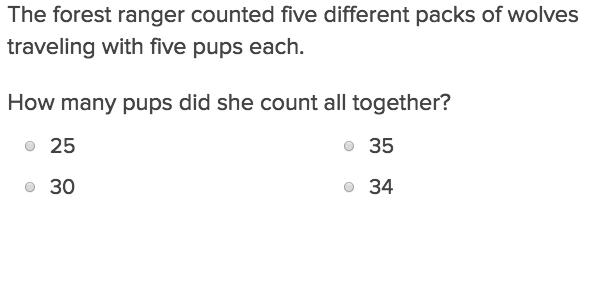Using equal groups, this exercise will help students just starting out with one digit multiplication.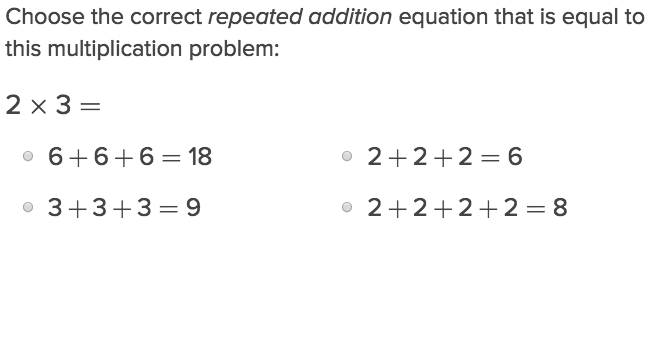This exercise teaches students a new trick by using repeated addition to achieve the same result as multiplication.

## One-Digit Multiplication with Skip-Counting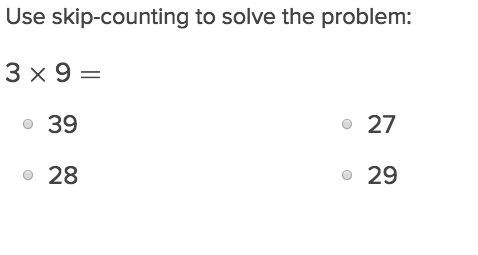Students can incorporate skip counting as a tool used in verifying their correct multiplication answers.

## Candy Shop Arrays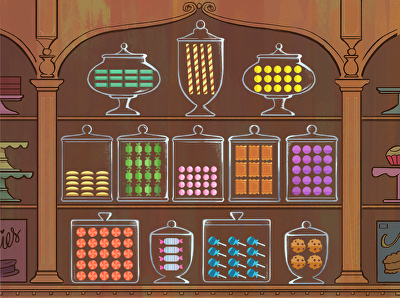Yum, candy! Make multiplication a little sweeter with this candy shop array. By listening to the narrator, second graders can find candy jars containing the correct multiplication array. To do so, they'll count rows and columns, gaining understanding of one-digit multiplication in the process. Also great for third graders, this math game is a tasty way for kids to practice multiplication.

## One-Digit Multiplication and Arrays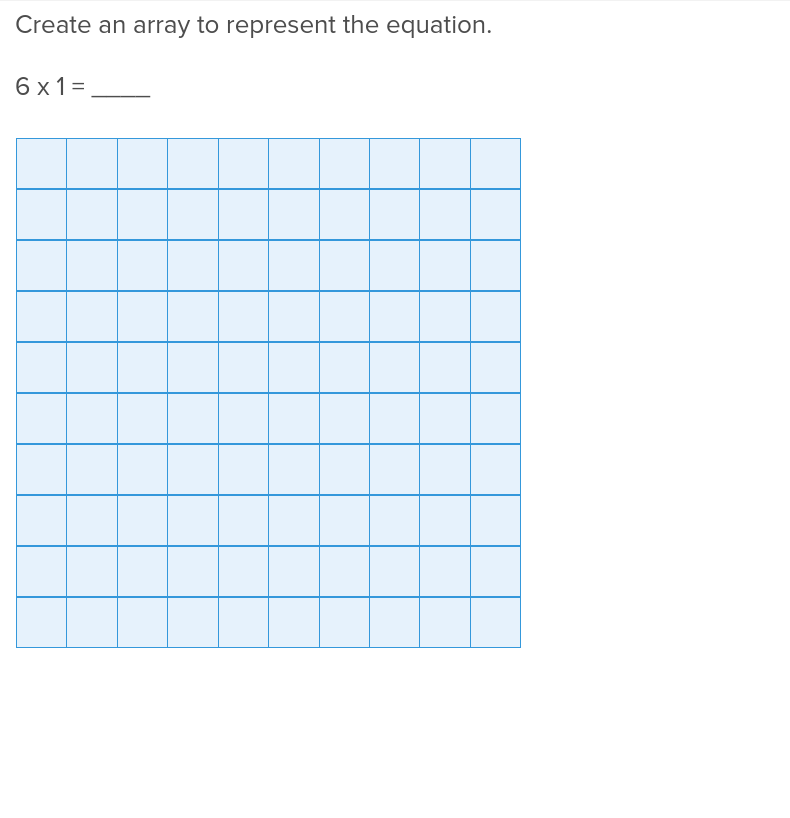Visual learners will appreciate the one digit arrays that make multiplication practice easier in this exercise.

## One-Digit Multiplication and the Commutative Property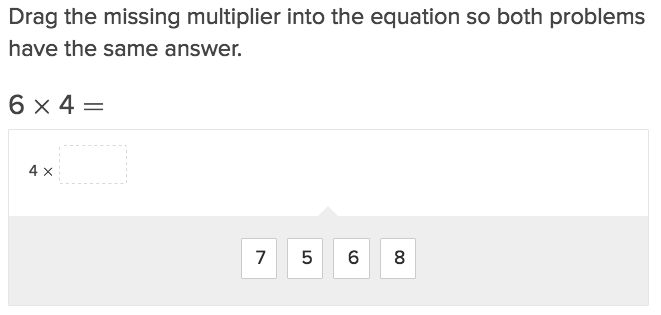Explain to students how the commutative property plays out in multiplication problems with this exercise.

## One-Digit Multiplication Using a Number Line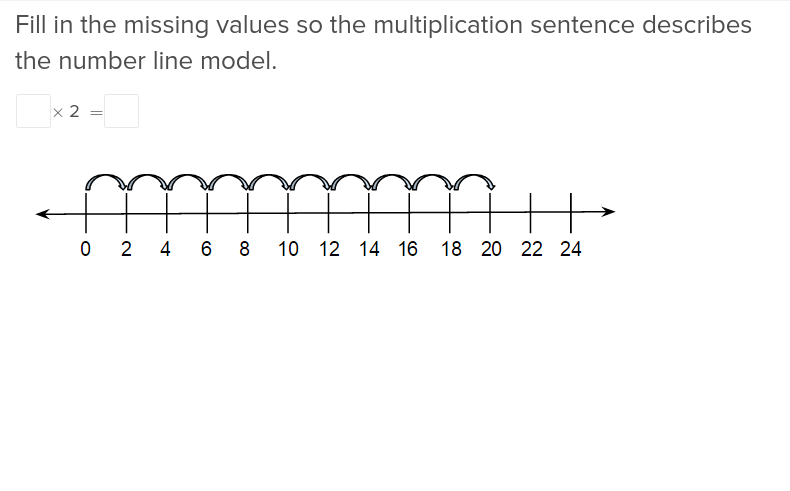Third grade multiplication is made easy with these exercises that have students utilize a number line and helpful hints.

## Pool Creation: Area Models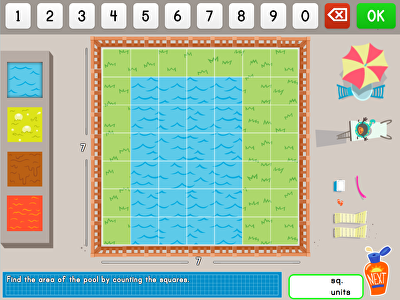Make a splash with multiplication! In this math game, kids calculate the area of a family's pool with an array. Perfect for practicing simple multiplication or repeated addition, this game helps players understand how to multiply using concrete manipulatives. In addition, kids are challenged to answer a word problem about fun pool add-ons, taking the math practice to the next level.

## One-Digit Multiplication with Area Models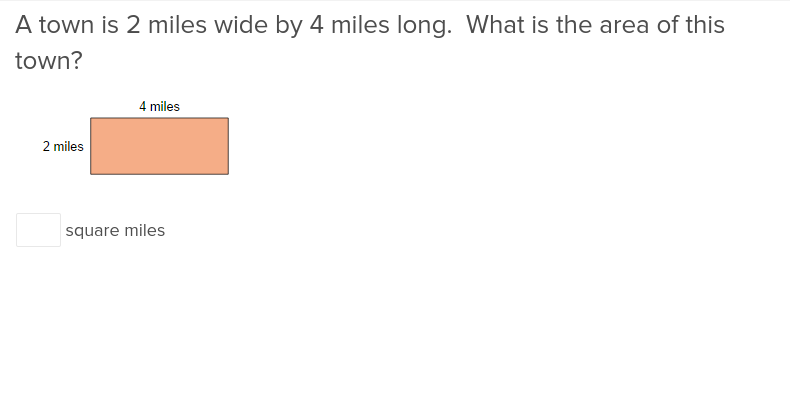Give your students the foot up they need in geometry with these exercises that have your third graders work forwards and backwards through area problems.

## Relating Area to Addition and Multiplication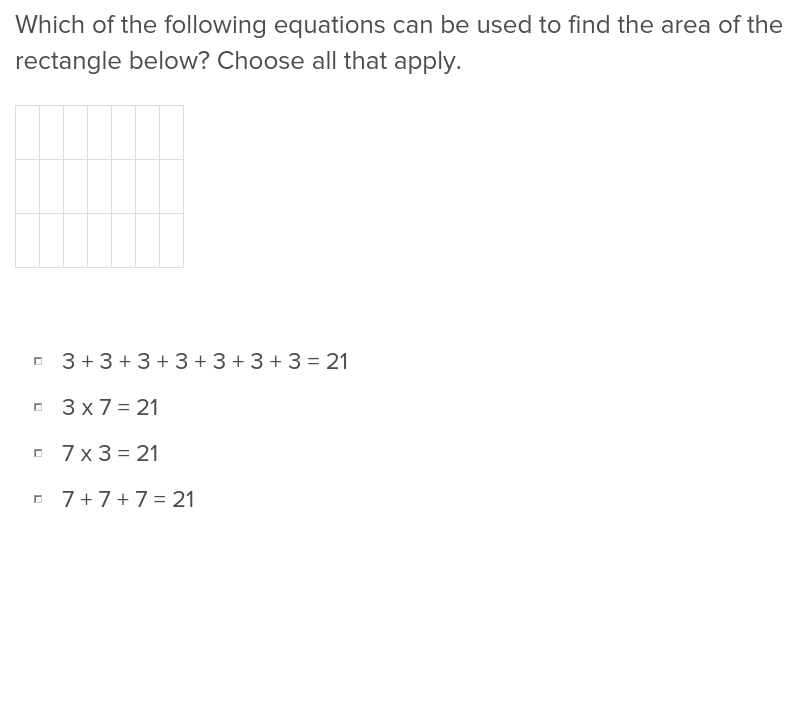Help students understand the relation between addition and multiplication, and the area of objects, with this easy to navigate exercise.

## Multiply by 1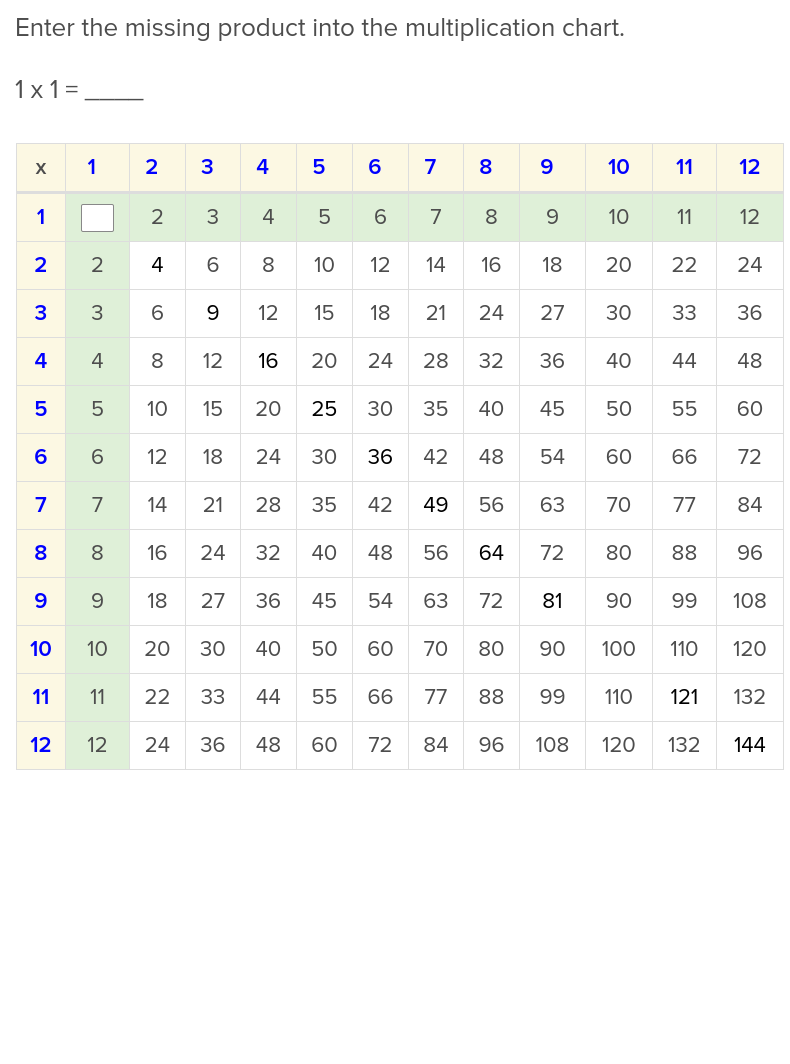A great starting point with teaching multiplication is having students learn facts about multiplying 1s.

## Multiply by 2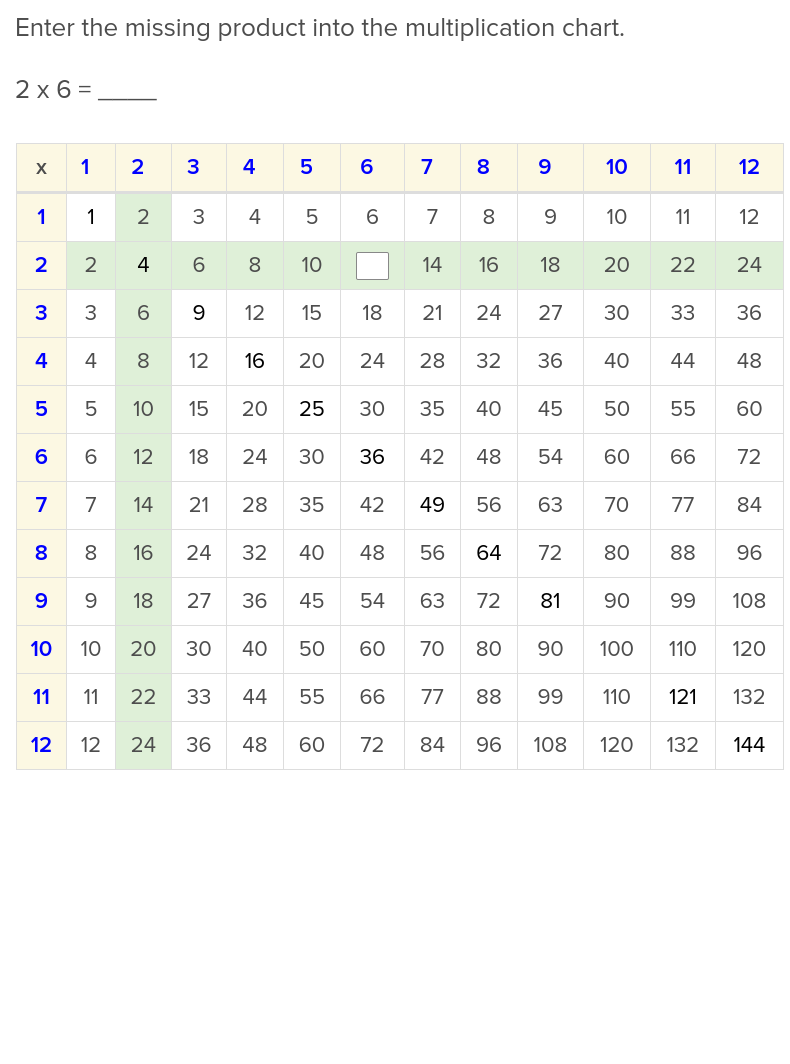Students who are attracted to the difference between odds and evens will like studying these facts about multiplying 2s.

## Multiply by 10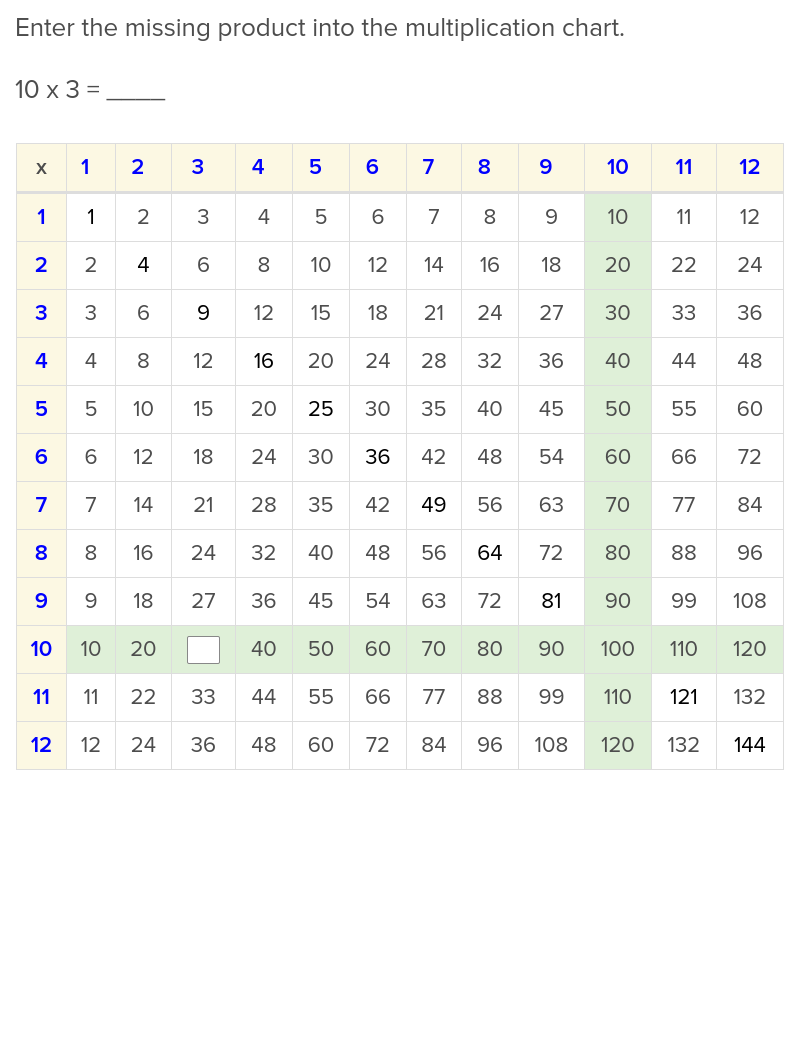Whether because it’s a double digit number or it presents round answers, students gravitate to learning facts about multiplying 10s.

Create new collection

0

### New Collection>

0 items

What could we do to improve Education.com?